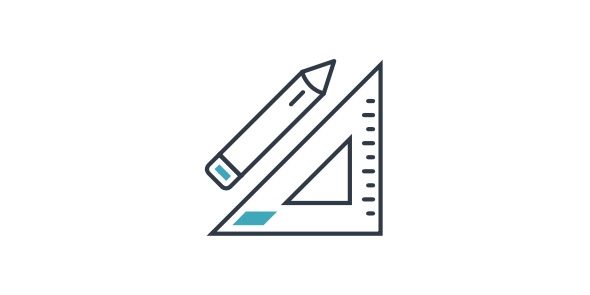# 7th Grade: Math Percentage Quiz!

12 Questions | Total Attempts: 1919SettingsThis is percentage practice for TABLE. You have 12 free response questions

• 1.
170% of 86
• 2.
10% of 61
• 3.
59% 0f 30=
• 4.
100% of 70
• 5.
90% of ___ =27
• 6.
180% 0f ____ =108
• 7.
90% of ____ =72
• 8.
80% of ____  =64
• 9.
____% of 190=171
• 10.
____% of 80=72
• 11.
____% of 130=78
• 12.
____% of 180 =54
Related Topics